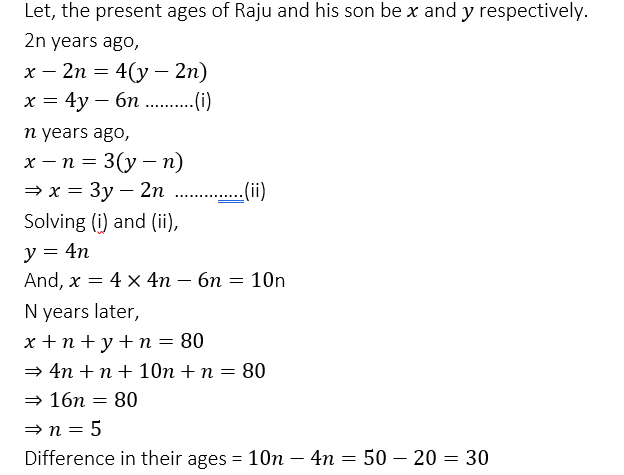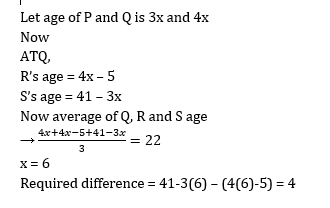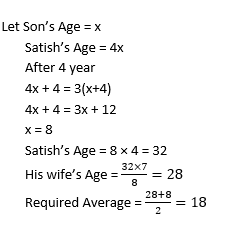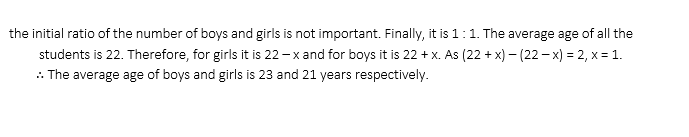# Age based Quant Quiz for SBI PO PRE 2019 (Day 16) | 19th February 2019

## Age based Quant Quiz for SBI PO PRE 2019 (Day 16)

Quant Quiz to improve your Quantitative Aptitude for SBI PO & SBI clerk exam quant, IBPS PO quant , IBPS Clerk quant , IBPS RRB quant, and other competitive exams.

Q1. The ratio of Radha’s and Ruchi’s ages is 9 : 4. If the difference between the present age of Radha and the age of Ruchi 5 year hence is 5 then what is the sum of the present ages of Radha and Ruchi?

A) 18 years

B) 16 years

C) 26 years

D) 32 years

E) None of these

Q2. 2n years ago, the age of Raju was four times that of his son and n years ago, the age of Raju was thrice that of his son. If n years later, the sum of the ages of Raju and his son will be 80 years, then the difference in the ages of Raju and his son is

A) 20 years

C) 40 years

D) 24 years

E) 30 years

E) 34 years

Q3. The sum of the ages of father and son is 50 years. Eight years ago, the product of their ages was two time the father’s age at that time, then the present ages (in years) of the father and son respectively are

A) 39,6

B) 35,10

C) 36,9

D) 40,10

E) None of these

Q4.  If age of P and R are added to twice the age of Q, the total becomes 59. It the ages of Q and R are added to thrice the age of P, the total become 68. And if the age of P is added to thrice the age of Q and thrice age of R, the total becomes 108, what is the total of ages of P, Q and R?

A) 45 years

B)  47 years

C) 49 years

D) 42 years

E)  44 years

Q5.  3 years ago Ambuj was thrice as old as Avinash. If he ratio of their present ages is 8 : 3 respectively, what is the difference between their present ages in years ?

A) 32 years

B) 30 years

C) 28 years

D) 35 years

E) None of these

Q6. The ratio of the present ages of a son and his father is 1 : 5 and that of his mother and father is 4 : 5. After 2 years the ratio of the age of the son to that of his mother becomes 3 : 10. What is the present age of the father ?

A) 30 years

B)  28 years

C) 37 years

E) None of these

Q7. Sweta and Neha profess to tell their present ages as 25 and 20 years respectively. (Not original age). Ratio of their original ages 5 year ago is 5 : 4. Sum of ages of both 5 years hence is 400/9  more than the sum of present ages of both professed by them. Find the sum of their present original age (in years)

A) 25

B) 35

C) 55

D) 40

E) 50

Q8.  Ratio of present age of P and Q is 3 : 4. Sum of the present age of S and P is 41. R is 5 year younger than Q. Average of present age of Q, R and S is 22. Find the difference of S and R’s age (in years)

A) 3

B) 2

C) 1

D) 5

E) 4

Q9. Satish’s age is 4 times the present age of his son’s age. After 4 year the age of satish become 3 time the age of his son’s age. If Satish’s wife age is 7/8 of his age then find out the average of present age of Satish’s wife and his son(in years)

A) 18

B) 20

C) 14

D) 16

E) 21

Q10.  In a company there are 252 employees, in which the ratio of the number of men and women is 2 : 1. Some more women are employed and the ratio of men and women becomes 1 : 1. The average age of all the employees is now 22 years and the average age of the women is 2 years less than the average age of the men. Find the average age (in years) of men and women.

A) 22, 20

B) 23, 21

C) 24, 22

D) 24, 22

E) 21, 23

## Solutions

Q1. Ans(C)

Ruchi’s age=4x
According to question
9x-(4x+5)=5
9x-4x=10
x=2
Ruchi’s age=4×2=8
Sum=26 years

Q2. Ans(E)Q3. Ans(D)Q4. Ans(E)Q5. Ans(B)Q6. Ans(E)Q7. Ans(C)Q8. Ans(E)Q9. Ans(A)Q10. Ans(B)## WhatsApp Group Join here

Mail us at : ambitiousbaba1@gmail.com